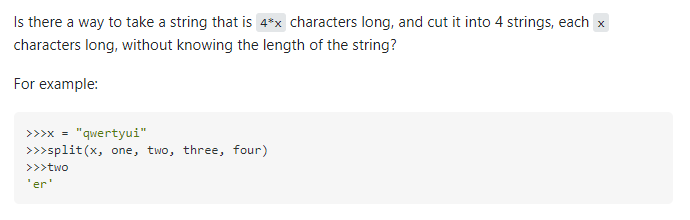# Python | Split String by Length

Summary: You can split a string by length by slicing the string into chunks of equal lengths. The indices to slice the string can be deduced using a list comprehension.

Minimal Example:

```text = "abcdefghijklmno"
print([text[i:i + 3] for i in range(0, len(text), 3)])

# OUTPUT: ['abc', 'def', 'ghi', 'jkl', 'mno']```

## Problem Formulation

📜Problem: Given a string, how will you split the string by length? Here’s a related question asked in stack overflow that perfectly demonstrates the given problem.

Related Question on StackOverflow:### Example

Consider that you have a string and you want to cut or split the string into chunks of substrings such that each substring has exactly five character. In other words each substring should have a length of five. Store each substring as an item in a list.

```# Given String
text = "threeseveneightfortyfifty"
# Output
['three', 'seven', 'eight', 'forty', 'fifty']```

Let’s dive into the solutions to the given problem.

## Method 1: Use a List Comprehension

The best way to deal with the given problem is to use a list comprehension that splits the string with the help of slicing.

Code:

```text = "threeseveneightfortyfifty"
print([text[i:i + 5] for i in range(0, len(text), 5)])

# OUTPUT: ['three', 'seven', 'eight', 'forty', 'fifty'] ```

Explanation: The for loop allows you to iterate over a range of numbers that are multiples of 5 (i.e. the size of the split substrings). You can use the values returned in each iteration to slice the strings into groups of substrings having 5 characters. Please follow the illustration given below to understand how this works –

Alternate Formulation using multi-line code: The solution given above can also be formulated using a for loop in multiple lines. Here’s how to do it:

```text = "threeseveneightfortyfifty"
res = []
for i in range(0, len(text), 5):
res.append(text[i:i + 5])
print(res)

# OUTPUT: ['three', 'seven', 'eight', 'forty', 'fifty']```

## Method 2: Use textwrap

Another workaround to solve the problem is to use the `textwrap` library’s `fill(text, width)` function, which allows you to wrap a string into equal width. This means the given string will be split (wrapped, to be exact) into numerous substrings of equal length/width. The output returned is a string object. Hence, you need to convert it to a list by using a normal split function.

Code:

```import textwrap

text = "threeseveneightfortyfifty"
x = textwrap.fill(text, 5).split()
print(x)

# OUTPUT: ['three', 'seven', 'eight', 'forty', 'fifty'] ```

## Method 3: Use regex

The regular expressions library leverages numerous functions to split a string in any given way. In this case, you can use the `compile` function of the regex library to solve the given problem.

Code:

```import re
text = "threeseveneightfortyfifty"
sre = re.compile(rf'(.{{{5}}})')
print([x for x in re.split(sre, text) if x])

# OUTPUT: ['three', 'seven', 'eight', 'forty', 'fifty'] ```

Do you want to master the regex superpower? Check out my new book The Smartest Way to Learn Regular Expressions in Python with the innovative 3-step approach for active learning: (1) study a book chapter, (2) solve a code puzzle, and (3) watch an educational chapter video.

## Method 4: Use a Generator

Another workaround to solve the problem is to use the `yield` keyword that creates a generator object and returns the split substrings one by one as items in a list.

Code:

```def split_len(s, n):
def _f(s, n):
while s:
yield s[:n]
s = s[n:]
return list(_f(s, n))

text = "threeseveneightfortyfifty"
chunks = 5
print(split_len(text, chunks))

# OUTPUT: ['three', 'seven', 'eight', 'forty', 'fifty'] ```

🌎Recommended Read: Yield Keyword in Python – A Simple Illustrated Guide

## Conclusion

We have successfully solved the given problem using different approaches. I hope this article helped you in your Python coding journey. Please subscribe and stay tuned for more interesting articles and solutions.

Happy Coding! 🙂

## Python Regex Course

Google engineers are regular expression masters. The Google search engine is a massive text-processing engine that extracts value from trillions of webpages.

Facebook engineers are regular expression masters. Social networks like Facebook, WhatsApp, and Instagram connect humans via text messages

Amazon engineers are regular expression masters. Ecommerce giants ship products based on textual product descriptions.  Regular expressions ​rule the game ​when text processing ​meets computer science.

If you want to become a regular expression master too, check out the most comprehensive Python regex course on the planet: